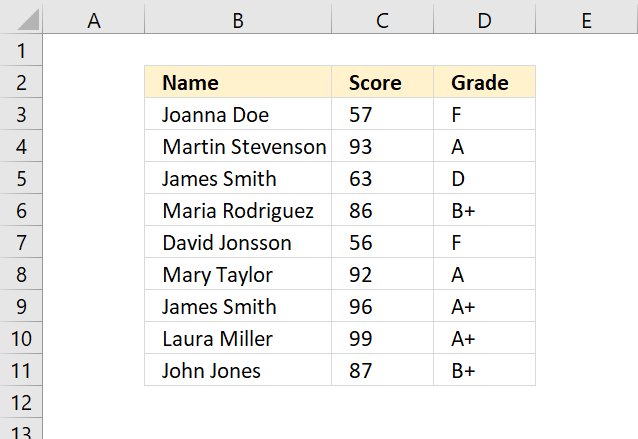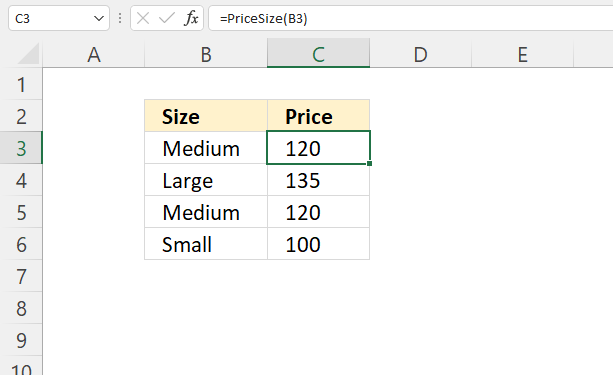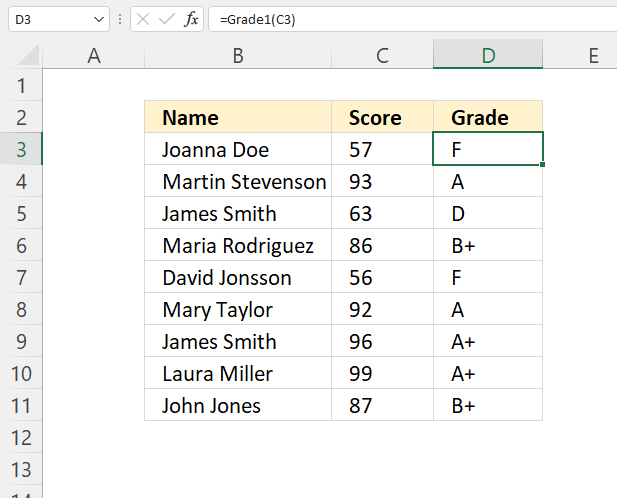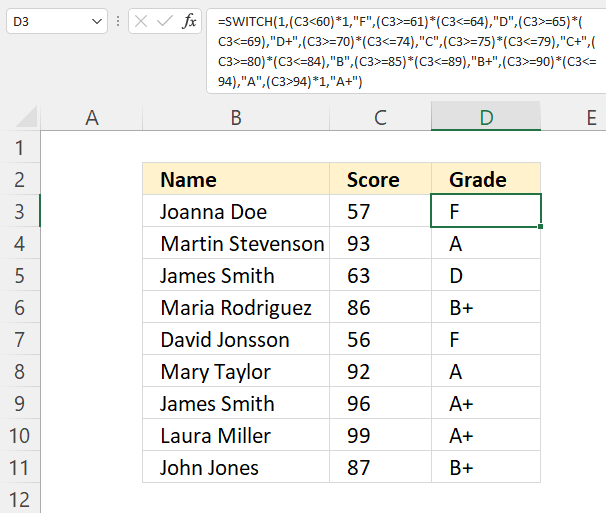Author: Oscar Cronquist Article last updated on July 04, 2022The SELECT CASE statement allows you to compare an expression to multiple values.

It is similar to the IF THEN ELSE statement except that the IF THEN ELSE statement can evaluate multiple expressions. The SELECT CASE statement evaluates one expression.

## 1. SELECT CASE statement Syntax

Select Case testexpression

[ Case expressionlist-n [ statements-n ]]
[ Case Else [ elsestatements ]]

End Select

## 2. SELECT CASE statement Parameters

 testexpression Required. Any expression: numeric expression - You can use variables, constants, keywords, and operators. The result is a number. string expression - Any expression that returns a string. expressionlist-n Required. True - ignore empty cells in the third argument. False - adds empty cells to the result. statements-n Required. The cell range you want to concatenate. elsestatements Optional. Up to 254 additional cell ranges.

## 3. SELECT CASE statement example 1

The User Defined Function above compares the score to several criteria and returns the corresponding grade.The UDF is entered in column D and takes the score from column C and returns the grade.

UDF in cell D3:

### VBA Code

```Function Grade(score)

Select Case score
Case Is < 60
Case 61 To 64
Case 65 To 69
Case 70 To 74
Case 75 To 79
Case 80 To 84
Case 85 To 89
Case 90 To 94
Case Else
End Select

End Function
```

## 4. SELECT CASE statement example 2Formula in cell D3:

=PriceSize(B3)

### VBA Code

```Function PriceSize(size)

Select Case size
Case Is = "Small"
PriceSize = 100
Case Is = "Medium"
PriceSize = 120
Case Is = "Large"
PriceSize = 135
End Select

End Function
```

## 5. SELECT CASE statement - VBA alternativeFormula in cell D3:

### VBA Code

```Function Grade1(score)

If score < 60 Then

ElseIf score <= 64 And score >= 61 Then

ElseIf score <= 65 And score >= 69 Then

ElseIf score <= 74 And score >= 70 Then

ElseIf score <= 79 And score >= 75 Then

ElseIf score <= 84 And score >= 80 Then

ElseIf score <= 89 And score >= 85 Then

ElseIf score <= 94 And score >= 90 Then

Else

End If

End Function
```

## 6. SELECT CASE statement - Excel Function alternativeFormula in cell D3:

=SWITCH(TRUE, C3<60, "F", C3<=64, "D", C3<=69, "D+", C3<=74, "C", C3<=79, "C+", C3<=84, "B", C3<=89, "B+", C3<=94, "A", C3>94, "A+")

### Get Excel *.xlsm file

How to use SELECT CASE statement.xlsm